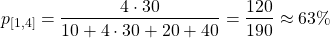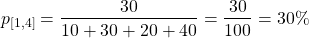# Logic Design - Constraint Types in SystemVerilog (part 1)

in STEMGeekslast year[Edit of Image1]

## Introduction

Hey it's a me again @drifter1!

Today we continue with the Logic Design series on SystemVerilog in order to talk about the various Constraint Types. The topic will be split into two parts.

So, without further ado, let's get straight into it!

## Simple Expressions

Now that we've seen how constraints are defined, we can cover the types of conditions and expressions that can be added to them.

First of all, a condition in a constraint can be an expression that contains a single relational operator `<`, `<=`, `>` or `>=`.

For example, considering an integer `val`. A simple constraint could be of the form:

``````constraint c_simple { 0 < val;  val < 32; }
``````

where `val` will have to be in the non-inclusive range (0, 32).

Additionally, assignments (`=`) are not allowed. Instead, the equivalence operator (`==`) has to be used.

For example, specifying that `val` has to be 12 is done as follows:

``````constraint c_equal { val == 12; }
``````

Such a constraint is more commonly added as an in-line constraint, in order to force a value to a variable.

## Set Membership (Inside Operator)

Specifying a range of values (or specific allowed values) is quite common, and so there's no need for two separate "relational" conditions to be defined as SystemVerilog provides the `inside` operator for that purpose. The `inside` operator can be used for specifying the allowed range or allowed values in general in the form of a set.

The complete range or set of values that a variable should satisfy is enclosed within `{}`. Each individual range or value is separated by a comma (`,`). So, it's possible to specify multiple ranges and values, in order to create the valid set.

### Range of Values

A range is specified within `[]` with the lower and upper limit separated by `:`.

For example, the range constraint specified previously can also be written as:

``````constraint c_range { val inside [1 : 31]; }
``````

Note that the range specified by the `inside` operator includes the lower and upper limits, and basically works like a `>=` and `<=`.

### Set of Values

A simple set constraint can be written as:

``````constraint c_set { val inside {2, 4, 8, 16}; }
``````

where `val` now would have to be either 2, 4, 8 or 16 for the condition to be satisfied.

### Inverted Inside Operator

The `inside` operator can also be inverted, by adding the negation operator `!` before the condition and enclosing the complete expression in parentheses.

For example, specifying that `val` should not be equal to 8 and 16 can be done as follows:

``````constraint c_iset { !(val inside {8, 16}); }
``````

## Weighted Distributions (Dist Operator)

SystemVerilog also provides weight-based randomization. Using the `dist` operator it's possible to specify which values (or ranges) should have a higher priority in randomization.

The weighted ranges and values are enclosed within `{}` and separated by comma (`,`).

### := Operator

Specifying the weight for a specific value is done using the `:=` operator. The same operator is also used when the weight should be the same for every single value in a range.

For example:

``````constraint c_weighted {
val dist {
0 := 10,
[1 : 4] := 30,
5 := 20,
6 := 40
};
}
``````

With this definition the weight of every single value in the range [1, 4] is 30, meaning that the probability of choosing a value in that range is quite high:### :/ Operator

Dividing the specified weight equally among the values in a range is done using the `:/` operator.

Let's tweak the previous example:

``````constraint c_weighted2 {
val dist {
0 := 10,
[1 : 4] :/ 30,
5 := 20,
6 := 40
};
}
``````

Now the probability of choosing a value in the range [1, 4]* is:So, each value in the range is of weight 30 / 4 = 7.5, instead of 30.

## RESOURCES:

### Images

Block diagrams and other visualizations were made using draw.io

## Previous articles of the series

### SystemVerilog

• From Verilog To SystemVerilog → Data Types, Arrays, Structures, Operators and Expressions
• Control Flow → Additional Procedural Blocks, Loops, Conditional Statements, Functions and Task Features
• Processes → Fork - Join in Verilog and SystemVerilog, Process Control (wait fork, disable fork)
• Events → Interprocess Communication, Events (Definition, Triggering, Waiting, Sequencing, Merging, as Arguments)
• Semaphores and Mailboxes → Semaphores (Creation, Methods), Mailboxes (Definition, Methods)
• Interfaces (part 1) → Interfaces (Definition, Port and Signal Lists, Instantiation), Modports
• Interfaces (part 2) → Parameters, Tasks and Functions (Importing, Exporting), Clocking Blocks (Input and Output Skews)
• Classes (part 1) → Classes (Definition, Constructor Function, Creating Objects, Accessing Class Members, Static and Constant Class Members, Arrays)
• Classes (part 2) → Copying Objects (Shallow and Deep Copy), Inheritance, Polymorphism, Virtual Methods
• Classes (part 3) → Parameterized Classes, Out-of-Block Method Declaration, Data Accessibility, Abstract / Virtual Classes
• Program Blocks → Design and Testbench, Program Block (Reactive Region, Allowed Constructs)
• Packages → Design Hierarchy, Packages (Definition, Importing, Definition Collision)
• Constraints and Randomization → Testing and Verification, Random Variables (Standard, Random-Cyclic), Randomize Method (Constraint and Random Mode, Pre / Post Randomize)
• Constraint Blocks → Constraint Blocks (Syntax, Rules), External (Explicit, Implicit), Static, Soft and In-Line Constraints

## Final words | Next up

And this is actually it for today's post!

Next time we will cover the remaining Constraint Types...

See Ya!Keep on drifting!

Posted with STEMGeeks